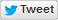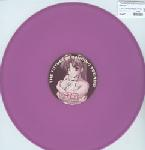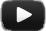0 (0 rates - 0 favorites)

# Radium - I Am The Hardstyle Pimp (TG Loves Small Tits Mix) lyrics

Ruler of the round now ruler of the breaks
[3x]

I'm the [3x]

Ruler of the round now ruler of the breaks
I'm the [3x]

Breaks [2x]
I'm the
[?x]

I'm the ruler of the round now ruler of the breaks [3x]
Like Puba Maxwell makes no mistakes
[3x]

I'm the ruler of the round now ruler of the breaks [3x]
I'm the ruler-ruler-ruler-breaks-breaks-breaks

I'm the ruler of the round now ruler of the breaks [3x]
Like Puba Maxwell makes no mistakes
[3x]

I'm the ruler of the round now ruler of the breaks [3x]

Future sound corporation
Engine sound

Keep it hardstyle

Ruler of the round now ruler of the breaks [3x]
Ruler of the round now ruler of the
Ruler of the round now ruler of the breaks
Ruler of the round now ruler of the
Ruler of the round now [?x]
Now of the breaks

Keep it hardstyle

I'm the ruler of the round now [2x]
I'm the ruler of the ruler of the ruler of the breaks

I'm the ruler of the round now [2x]
I'm the ruler of the breaks

I'm the ruler of the round now [2x]
I'm the ruler of the ruler of the ruler of the breaks
[3x]

I'm the ruler of the round now [2x]
Ru-ru-ru-breaks

I'm the ruler of the round now [2x]
I'm the ruler of the ruler of the ruler of the breaks
[?x]

Ru-ru-ru-ru-breaks• I Am The Hardstyle Pimp

• Year
• 2004

• Genre

• Source / Sample

• Submitted
• 1,077 views

Top achievedBETA
#3 Daily HardstyleRegister or login to comment on lyrics
• Rate tracks, request lyrics you don't understand, no ads, hide genres you don't like, etc.
Register